# Classifying space

The classifying space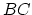of a category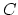is the geometric realization of the nerve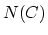. That is,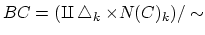where the equivalence relation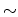glues the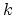-simplices togetehr as specified by the face and degeneracy maps of. For a group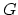, we can consider the category with a single object and morphisms given by elements of; in this case, this construction recovers the Borel construction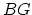. More generally, given a groupacting on a space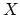, we can construct a (topological) category whose objects are given by points inand whose morphisms are given by elements of. The classifying space of this category is the homotopy quotient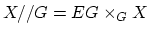. Ifis a strict symmetric monoidal category thenwill be an infinite loop space.

Note that in algebraic geometry,'' often refers to the stack-theoretic quotient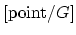.

Jeffrey Herschel Giansiracusa 2005-06-27